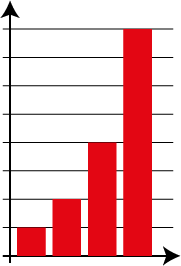Home > Reasoning > Big ideas > If-then > Proportional reasoning

# Proportional reasoning

One of the big ideas of fractions is the concept of a fraction as a ratio. That is, the size of a fraction can be thought of as the relationship between its numerator and denominator.

Ratios and proportions involve multiplicative comparisons.

Quantities can be compared and the value of one quantity can be predicted based on the value of another. In recipes for example, if one quantity is doubled then the other ingredients have to be doubled.Doubling quantities.

Proportional reasoning involves the consideration of numbers in relative terms and the use of multiplicative relationships.

While proportional reasoning is commonly taught in secondary mathematics classrooms, research shows that primary students can understand the basic concepts well.

Students continue to use proportional thinking when they work with scale diagrams, simultaneous equations, trigonometry and other topics, as well as in everyday problem solving.

The articleon the AAMT website Facilitating the Development of Proportional Reasoning Through Teaching Ratio identifies 'points of growth' that give teachers a framework for understanding students' thinking in terms of proportional reasoning.